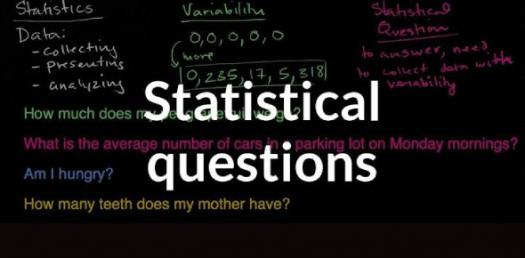# Basic Questions On AP Statistics! Quiz

5 Questions | Total Attempts: 528Settings.

Related Topics
• 1.
Two friends, Tom and Janice, have cars in desperate need of repair.  On any given day, the probability that Tom's car will break down is 0.5, the probability that Janice's car will break down is 0.5, and the probability that both cars will break down is 0.3.  What is the probability that Tom and Janice's car will break down?
• A.

1.3

• B.

1.0

• C.

0.7

• D.

0.4

• E.

0.2

• 2.
Summary statistics are calculated for a data set that includes an outlier.  If the outlier is removed, which summary statistic would be least affected?
• A.

Mean

• B.

Median

• C.

Range

• D.

Standard deviation

• E.

Variance

• 3.
Mr. B teaches two periods of English.  He has 38 seniors in one period and 24 juniors in the other period.  The overall mean for both periods combined on their Fall final grades was 87.  If the junior period had a mean of 92, what was the approximate mean for the senior period?
• A.

82.6

• B.

83.8

• C.

89.5

• D.

87.0

• E.

90.4

• 4.
In a game of chance, three fair coins are tossed simultaneously.  If all three coins are heads, then the player wins \$15.  If all three coins show tails, then the player wins \$10.  If it costs \$5 to play the game, what is the players expected gain or loss at the end of two games?
• A.

The player can expect to gain \$15 after two games.

• B.

The player can expect to gain \$1.88 after two games.

• C.

The player can expect to gain \$3.75 after two games.

• D.

The player can expect to lose \$1.88 after two games.

• E.

The player can expect to lose \$3.75 after two games.

• 5.
Which of the following is a true statement about experimental design?
• A.

Replication is a key component in experimental design. Thus, an experiment must be repeated many times before publicizing the results.

• B.

Control is a key component in experimental design. Thus, a control group that receives a placebo is a requirement for experimentation.

• C.

Randomization is a key component in experimental design. Randomization is used to spread uncontrolled variability among treatment groups.

• D.

Blocking eliminates the effects of all lurking variables.

• E.

The placebo effect is a concern for all experiments.